# Introduction - How to use EcoDiet

#### 2023-01-06

EcoDiet is a new tool for assimilating data in food-web studies. The goal of the package is to quantify trophic interactions between food-web components by combining stomach content analyses and biotracers in a Bayesian hierarchical model. To do so, the model simultaneously estimates a probabilistic topology matrix of the trophic network and the diet matrix. The topology matrix is a probabilistic description of “who eats whom” in the food web nad is composed of the probabilities that trophic links exist between food-web components, i.e. the probabilities $$\eta$$ that any prey is eaten by a given predator. The diet matrix is estimated conditionally to this topology and contains all diet proportions $$\Pi$$, hence it expresses the contribution (in %) of any prey to a given predator’s diet.

The full EcoDiet model and its application to real and simulated datasets are described in Hernvann et al. 2022, Ecol Appl. Use citation("EcoDiet") to get the full reference.

Several options are available within the present package:

• Default option - only stomach content and biotracer data are integrated into EcoDiet.

• Literature option - stomach content and biotracer data are integrated and results from expert knowledge or the literature are used to formulate informative priors on the topology and diet matrices.

The example showcased here is an artificial dataset, created to be simple to visualize and understand. In this example as in Hernvann et al. 2022, Ecol Appl the biotracers used are stable isotopes but EcoDiet could be used to treat other analyses (e.g., fatty acids, specific-compounds stable isotopes).

Before running the following code, please ensure that EcoDiet and the required tools are correctly installed and set up on your computer. Instructions can be found in the README’s instructions. If you’re all set, let’s load the EcoDiet package:

library(EcoDiet)

To apply EcoDiet to your own data, your stomach content, biotracer and literature data should be in a specific format, similar to those of following example. This data can for instance be imported into R using .csv files:

example_stomach_data <- read.csv("./data/my_stomach_data.csv")
example_biotracer_data <- read.csv("./data/my_biotracer_data.csv")

Here we will demonstrate how EcoDiet works on a simulated dataset stored within the EcoDiet package.

### Stomach content data

The stomach content table gathers the sum of occurrences of each prey trophic group in the analyzed stomachs of each consumer trophic group. The first column of the table contains the names of the prey trophic groups and the headers of the following columns contain the names of all the predator trophic groups. The last row of the table should be named “full”, and indicates how many (non-empty) stomachs have been analyzed for each trophic group.

example_stomach_data <- read.csv(system.file("extdata", "example_stomach_data.csv",
package = "EcoDiet"))
knitr::kable(example_stomach_data)
X huge large medium small
huge 0 0 0 0
large 11 0 0 0
medium 9 22 0 0
small 0 3 16 0
full 19 24 17 0

In this example, for the “huge” animals, 19 stomachs were analyzed and contained remainings. Among these stomachs, 17 contained “large” animal remainings and 12 contained “medium” animal remainings.

If some trophic groups of the studied food web don’t have associated stomach content analyses, you should add a line in this table indicating the name of this group and 0 in the other columns (it is the case here for “small” animals that are at the base of the trophic network).

### Biotracer data

The Biotracer table contains the analyses of different tracer concentrations. Each line of the table represents one individual on which were conducted biotracer analyses for various elements (here, stable isotope analyses for carbon and nitrogen). The first column of the table should be called “group” and contain name of the trophic group the individual belongs. The rest of the table contains the measures, one column corresponding to one biotracer.

example_biotracer_data <- read.csv(system.file("extdata", "example_biotracer_data.csv",
package = "EcoDiet"))
knitr::kable(example_biotracer_data)
group d13C d15N
medium -15.64284 13.840550
medium -15.56745 12.600720
medium -16.44420 14.037290
medium -17.16711 13.133810
small -17.95860 10.443507
small -16.56196 10.789742
small -17.04855 11.236032
small -17.14060 8.976896
small -16.23605 8.580734
large -15.24835 16.433410
large -14.03405 16.299340
large -16.76164 17.009600
large -16.53439 15.878830
large -14.93301 16.633490
huge NA NA

All the trophic groups of the studied food web should be present in this biotracer table. Thus, if biotracer analyses are missing for one given trophic group you still have to enter one line indicating the name of the trophic group and NA in the following columnds (as it is the case for the “huge” group).

The biotracers like stable isotope analyses are integrated in the model based on the trophic enrichment concept. Thus, for each biotracer informed, you also need to define the corresponding trophic discrimination factors corresponding to your the biotracers. In this example, we use common trophic discrimination factors for carbon and nitrogen:

trophic_discrimination_factor = c(0.8, 3.4)

## 2. Preprocess your data without literature data (Default option)

If you have data extracted from the literature, skip this and go to section 3.

Read this section if you don’t want to integrate information from the literature / expert knowledge. This is specified using the literature_configuration argument.

literature_configuration <- FALSE

### Preprocess the data

The preprocess_data function checks and rearranges the input data into a specific format that is read when running the EcoDiet model:

data <- preprocess_data(stomach_data = example_stomach_data,
biotracer_data = example_biotracer_data,
trophic_discrimination_factor = c(0.8, 3.4),
literature_configuration = literature_configuration
)
#> The model will investigate the following trophic links:
#>        huge large medium small
#> huge      0     0      0     0
#> large     1     0      0     0
#> medium    1     1      0     0
#> small     0     1      1     0

Error messages will appear if the input data hasn’t been provided in the expected shape. Please read carefully the error message and rearrange your data in the correct format.

Stomach content dataset dominated by numerous small values of occurrence might be problematical as to few groups could be identified as potential prey. To avoid such situations, you can choose to upscale the stomach content data so that occurrences are artificially increased but their relative importance are conserved. This is performed by specifying rescale_stomach = TRUE when preprocessing the data.

data <- preprocess_data(biotracer_data = example_biotracer_data,
trophic_discrimination_factor = c(0.8, 3.4),
literature_configuration = literature_configuration,
stomach_data = example_stomach_data,
rescale_stomach = TRUE)
#> The model will investigate the following trophic links:
#>        huge large medium small
#> huge      0     0      0     0
#> large     1     0      0     0
#> medium    1     1      0     0
#> small     0     1      1     0

## 3. Preprocess your data with literature data (Literature option)

If you don’t have data extracted from the literature, skip this and go to section 4.

Read this section if you do want to integrate information from the literature / expert knowledge. This is specified using the literature_configuration argument.

literature_configuration <- TRUE

### Define the priors

A literature diet table is used to set priors on the trophic link probabilities $$\eta$$ and the diet proportions $$\Pi$$. This table is similar to the stomach contents table, as all trophic groups must be included in the columns and rows. The numbers are the average diet proportions found in the literature. Here, the selected studies have identified that “huge” animals eat equally “large” and “medium” animals (thus the 0.5 and 0.5 numbers in the first column). The proportions for a given predator (i.e., within a given column) must sum to 1. The “small” animals are at the base of the ecosystem, so the column is filled with zeros.

The last row of the table corresponds to the literature pedigree score. This score (a number from 0 to 1) quantifies the literature reliability on each predator’s diet. Here the dietary proportions from the literature are used to produce reliable estimates for the “huge” animals, e.g., the pedigree score associated is high (0.9). On the contrary, the diet proportions for the “medium” animals come from an older article focusing on a very different ecosystem so estimates produced are less reliable, e.g, the pedigree score is low (0.2). The pedigree score for the “small” animals is set at 1, because this group eats nothing. For more details please read the reference article.

example_literature_diets_path <- system.file("extdata", "example_literature_diets.csv",
package = "EcoDiet")
knitr::kable(example_literature_diets)
X huge large medium small
huge 0.0 0.0 0.0 0
large 0.5 0.0 0.0 0
medium 0.5 0.7 0.0 0
small 0.0 0.3 1.0 0
pedigree 0.9 0.6 0.2 1

This summary of the literature data will be used to formulate:

1. The priors on the topology matrix’s $$\eta$$s. If a given literature diet proportion is zero, the corresponding prior Beta distribution of $$\eta$$ will be shifted toward 0. If the proportion is positive, the distribution will be shifted toward 1.

2. The priors on the diet matrix’s $$\Pi$$s. The literature diet proportions are entered as the hyperparameters of the prior Dirichlet distribution of $$\Pi$$.

The Pedigree scores are used to determine the priors’ precision. Other parameters can be used to adjust the prior distributions:

• the nb_literature parameter. The higher the number, the stronger the weight will be of the literature in the final inference on $$\eta$$. Setting this parameter to 10 is like saying that the prior from the literature will weigh as much as the additional data from 10 stomachs. Thus for any particular application, nb_literature should be set to a value smaller than the sample size in the available stomach content data.
nb_literature = 10
• the literature_slope parameter (a value between 0 and 1). The higher the number, the stronger the weight will be of the literature in the final inference on $$\Pi$$. You should set this value depending on the value of your data (number of biotracers, etc).
literature_slope = 0.5

### Preprocess the data

The preprocess_data function then checks and rearranges the data in a specific format so that the EcoDiet model can be run:

data <- preprocess_data(biotracer_data = example_biotracer_data,
trophic_discrimination_factor = c(0.8, 3.4),
literature_configuration = literature_configuration,
stomach_data = example_stomach_data,
literature_diets = example_literature_diets,
nb_literature = 10,
literature_slope = 0.5)
#> The model will investigate the following trophic links:
#>        huge large medium small
#> huge      0     0      0     0
#> large     1     0      0     0
#> medium    1     1      0     0
#> small     0     1      1     0

If any error appears, it means your data is not in the correct format. Please read the error message and try to rearrange the data in the correct format.

If you have a lot of small values in the stomach occurences, you can choose to upscale the stomach content data:

data <- preprocess_data(biotracer_data = example_biotracer_data,
trophic_discrimination_factor = c(0.8, 3.4),
literature_configuration = literature_configuration,
stomach_data = example_stomach_data,
rescale_stomach = TRUE,
literature_diets = example_literature_diets,
nb_literature = 10,
literature_slope = 0.5)
#> The model will investigate the following trophic links:
#>        huge large medium small
#> huge      0     0      0     0
#> large     1     0      0     0
#> medium    1     1      0     0
#> small     0     1      1     0

## 4. Plot the data and the priors

You can visualize your data with the plot_data function:

plot_data(biotracer_data = example_biotracer_data,
stomach_data = example_stomach_data)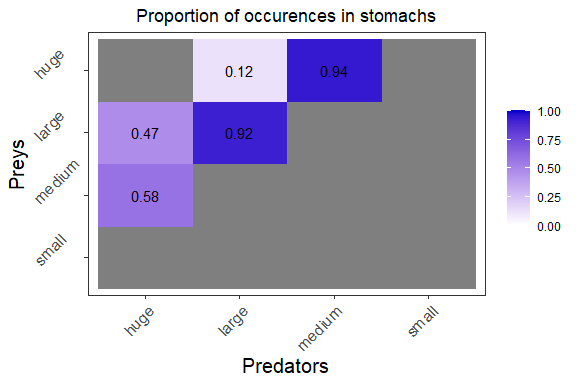#> Warning: Use of biotracer_data$group is discouraged. Use group instead.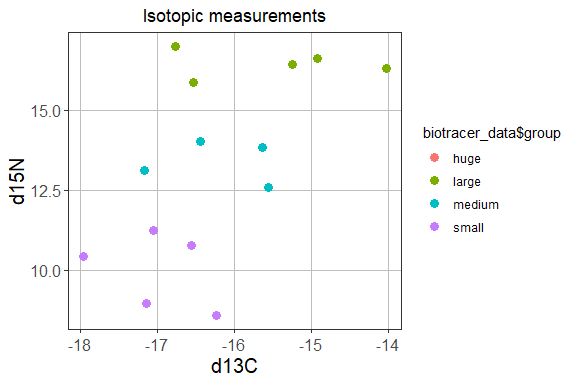You can save the figures as PNG in the current folder using: plot_data(biotracer_data = example_biotracer_data, stomach_data = example_stomach_data, save = TRUE, save_path = ".") Whether the priors are non-informative or informed by the literature, you can plot the mean of the prior distributions for the trophic link probabilities $$\eta$$ and the diet proportions $$\Pi$$: plot_prior(data, literature_configuration)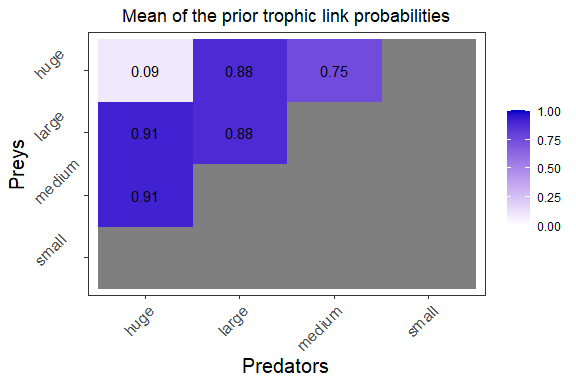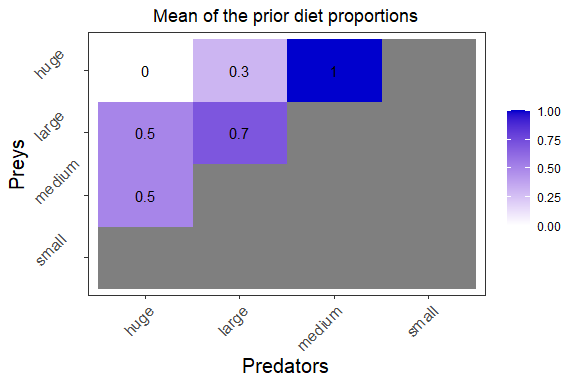You can also see the prior distributions for one trophic group (or predator): plot_prior(data, literature_configuration, pred = "huge")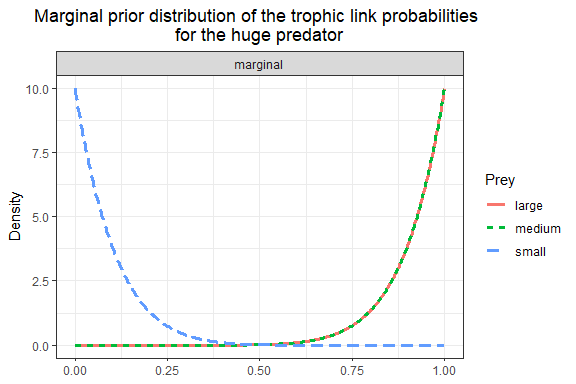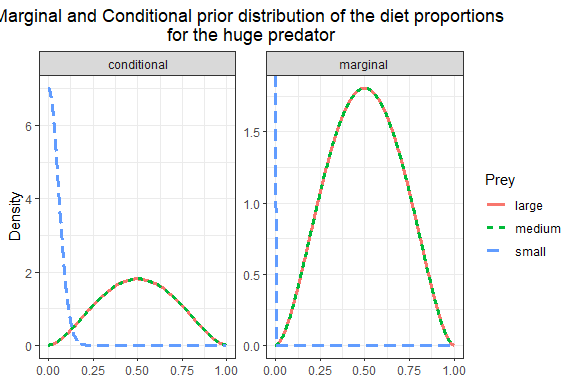This way, you can change the prior parameters and see how it affects the prior distributions. Here, we will change the nb_literature parameter from 10 to 2: data <- preprocess_data(biotracer_data = example_biotracer_data, trophic_discrimination_factor = c(0.8, 3.4), literature_configuration = literature_configuration, topology = topology, stomach_data = example_stomach_data, literature_diets = example_literature_diets, nb_literature = 2, literature_slope = 0.5) #> The model will investigate the following trophic links: #> huge large medium small #> huge 0 0 0 0 #> large 1 0 0 0 #> medium 1 1 0 0 #> small 1 1 1 0 plot_prior(data, literature_configuration, pred = "huge", variable = "eta")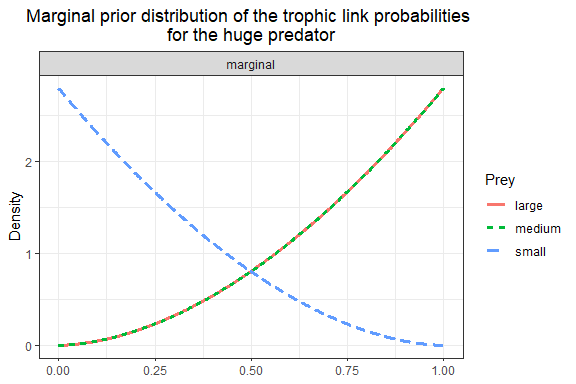## 5. Run the model The write_model function writes the model in the BUGS syntax. Here You need to specify whether you want to use or not priors form the literature since the model structure will be affected by this choice. The model is written as a .txt for which you should specify a path and name through the file.name argument. To see the written model, you can turn on visualization using print.model=TRUE or opening the saved .txt file filename <- "mymodel.txt" write_model(file.name = filename, literature_configuration = literature_configuration, print.model = F) First, run the model as a test (i.e., low adaption phase and low number of iterations) to check that the model is compiling properly. Specifying run_param="test" will by default run the model with nb_iter=1000 and nb_burnin=500. mcmc_output <- run_model(filename, data, run_param = "test") #> #> Processing function input....... #> #> Done. #> #> Compiling model graph #> Resolving undeclared variables #> Allocating nodes #> Graph information: #> Observed stochastic nodes: 20 #> Unobserved stochastic nodes: 41 #> Total graph size: 393 #> #> Initializing model #> #> Adaptive phase, 500 iterations x 3 chains #> If no progress bar appears JAGS has decided not to adapt #> #> #> Burn-in phase, 500 iterations x 3 chains #> #> #> Sampling from joint posterior, 500 iterations x 3 chains #> #> #> Calculating statistics....... #> Warning in doTryCatch(return(expr), name, parentenv, handler): At least one Rhat #> value could not be calculated. #> #> Done. #> #> /!\ Convergence warning: #> Out of the 13 variables, 3 variables have a Gelman-Rubin statistic > 1.1. #> You may consider modifying the model run settings. #> The variables with the poorest convergence are: PI[3,1], PI[4,1], PI[2,1]. #> JAGS output for model 'mymodel.txt', generated by jagsUI. #> Estimates based on 3 chains of 1000 iterations, #> adaptation = 500 iterations (sufficient), #> burn-in = 500 iterations and thin rate = 1, #> yielding 1500 total samples from the joint posterior. #> MCMC ran for 0.018 minutes at time 2023-01-06 12:43:17. #> #> mean sd 2.5% 50% 97.5% overlap0 f Rhat n.eff #> eta[2,1] 0.596 0.103 0.378 0.602 0.780 FALSE 1 1.001 1500 #> eta[3,1] 0.505 0.103 0.310 0.505 0.707 FALSE 1 1.005 357 #> eta[4,1] 0.044 0.042 0.001 0.032 0.151 FALSE 1 1.003 1500 #> eta[3,2] 0.888 0.060 0.743 0.897 0.977 FALSE 1 1.000 1500 #> eta[4,2] 0.190 0.073 0.073 0.184 0.351 FALSE 1 1.000 1500 #> eta[4,3] 0.898 0.067 0.734 0.912 0.989 FALSE 1 1.001 1296 #> PI[2,1] 0.462 0.343 0.000 0.471 0.983 FALSE 1 1.156 17 #> PI[3,1] 0.337 0.356 0.000 0.188 1.000 FALSE 1 1.534 7 #> PI[4,1] 0.201 0.243 0.000 0.110 0.877 FALSE 1 1.205 15 #> PI[3,2] 0.942 0.120 0.605 0.992 1.000 FALSE 1 1.054 1500 #> PI[4,2] 0.058 0.120 0.000 0.008 0.395 FALSE 1 1.054 1500 #> PI[4,3] 1.000 0.000 1.000 1.000 1.000 FALSE 1 NA 1 #> deviance 75.844 6.274 65.544 74.953 90.129 FALSE 1 1.020 124 #> #> **WARNING** Rhat values indicate convergence failure. #> Rhat is the potential scale reduction factor (at convergence, Rhat=1). #> For each parameter, n.eff is a crude measure of effective sample size. #> #> overlap0 checks if 0 falls in the parameter's 95% credible interval. #> f is the proportion of the posterior with the same sign as the mean; #> i.e., our confidence that the parameter is positive or negative. #> #> DIC info: (pD = var(deviance)/2) #> pD = 19.4 and DIC = 95.231 #> DIC is an estimate of expected predictive error (lower is better). The low numbers won’t be enough to achieve a satisfactory model convergence. You should progressively increase the number of adaptation steps nb_adapt and of iterations nb_iter while checking the “Convergence warnings” message. You can specify these model run parameters by specifying run_param=list(nb_iter=iter, nb_burnin=burnin, nb_thin=thin). There are also a couple of default run parameters (“very short”,“short”,“normal”,“long”,“very long”) that you can use in the with run_param and learn about by calling ?run_param Be aware that, depending on your data and especially the number of trophic groups and investigate trophic interactions, the model can take hours or days to run. mcmc_output <- run_model(filename, data, run_param=list(nb_iter=10000, nb_burnin=5000, nb_thin=5)) mcmc_output <- run_model(filename, data, run_param=list(nb_iter=50000, nb_burnin=25000, nb_thin=25)) mcmc_output <- run_model(filename, data, run_param=list(nb_iter=100000, nb_burnin=50000, nb_thin=50)) mcmc_output_example <- run_model(filename, data, run_param=list(nb_iter=50000, nb_burnin=25000, nb_thin=25)) Note that for much complex case studies, the dimension of the model may be considerable hence the time required by the runs may be dramatically long. In that context, while analyzing your results carefully, you might be willing to use your model outputs if a only a very limited number of variables don’t reach convergence after especially long runs. Once a model reaches convergence objectives, don’t forget to save it on your machine. save(mcmc_output_example, file = "./data/mcmc_output_example.rda") ### Diagnoses Here we provide the diagnose_model function to perform simple diagnoses on the EcoDiet run. It displays the number of variables for which the Gelman-Rubin test remains higher than different thresholds, highlighting also the 10 worst variables in terms of convergence. The object created contains these values for all the model variables. Gelman_model <- diagnose_model(mcmc_output_example) #> #> ################################################################################ #> # Gelman-Rubin Diagnostic #> ################################################################################ #> In EcoDiet, we recommend a that Gelman diagnostic is < 1.1 #> Out of 11 variables: #> 0 > 1.01 #> 0 > 1.05 #> 0 > 1.1 #> The worst variables are: #> Point est. Upper C.I. #> PI[2,1] 1.006849 1.025859 #> PI[3,1] 1.006849 1.025859 #> PI[3,2] 1.004532 1.009351 #> PI[4,2] 1.004532 1.009351 #> eta[3,1] 1.001312 1.004666 #> eta[4,3] 1.001031 1.002551 #> eta[4,2] 1.000933 1.004568 #> eta[2,1] 1.000618 1.001747 #> eta[3,2] 1.000299 1.002006 #> deviance 1.000124 1.001733 print(Gelman_model) #>$gelman
#>          Point est. Upper C.I.
#> eta[2,1]   1.000618   1.001747
#> eta[3,1]   1.001312   1.004666
#> eta[3,2]   1.000299   1.002006
#> eta[4,2]   1.000933   1.004568
#> eta[4,3]   1.001031   1.002551
#> PI[2,1]    1.006849   1.025859
#> PI[3,1]    1.006849   1.025859
#> PI[3,2]    1.004532   1.009351
#> PI[4,2]    1.004532   1.009351
#> deviance   1.000124   1.001733

The function can also be used to produce diagnostic plots and save them as a .pdf file. Complex models will have so many variables that the process can be very long so the user can also specify the variable for which graphs should be produced and stored.

diagnose_model(mcmc_output_example, var.to.diag = "all", save = TRUE)

## 6. Plot and save the results

The object output by the run_model function contains all MCMC samples, summary statistics and the configuration of the run:

str(mcmc_output_example)
#> List of 24
#>  $sims.list :List of 3 #> ..$ eta     : num [1:3000, 1:4, 1:3] NA NA NA NA NA NA NA NA NA NA ...
#>   ..$PI : num [1:3000, 1:4, 1:3] NA NA NA NA NA NA NA NA NA NA ... #> ..$ deviance: num [1:3000] 73.8 89.2 75.8 80.1 82 ...
#>  $mean :List of 3 #> ..$ eta     : num [1:4, 1:3] NA 0.699 0.63 NA NA ...
#>   ..$PI : num [1:4, 1:3] NA 0.51 0.49 NA NA ... #> ..$ deviance: num 75.8
#>  $sd :List of 3 #> ..$ eta     : num [1:4, 1:3] NA 0.0824 0.0875 NA NA ...
#>   ..$PI : num [1:4, 1:3] NA 0.378 0.378 NA NA ... #> ..$ deviance: num 6.1
#>  $q2.5 :List of 3 #> ..$ eta     : num [1:4, 1:3] NA 0.532 0.446 NA NA ...
#>   ..$PI : num [1:4, 1:3] NA 4.57e-11 1.09e-11 NA NA ... #> ..$ deviance: num 65.7
#>  $q25 :List of 3 #> ..$ eta     : num [1:4, 1:3] NA 0.644 0.572 NA NA ...
#>   ..$PI : num [1:4, 1:3] NA 0.1275 0.0806 NA NA ... #> ..$ deviance: num 71.5
#>  $q50 :List of 3 #> ..$ eta     : num [1:4, 1:3] NA 0.705 0.634 NA NA ...
#>   ..$PI : num [1:4, 1:3] NA 0.503 0.497 NA NA ... #> ..$ deviance: num 75.2
#>  $q75 :List of 3 #> ..$ eta     : num [1:4, 1:3] NA 0.758 0.69 NA NA ...
#>   ..$PI : num [1:4, 1:3] NA 0.919 0.873 NA NA ... #> ..$ deviance: num 79.6
#>  $q97.5 :List of 3 #> ..$ eta     : num [1:4, 1:3] NA 0.848 0.794 NA NA ...
#>   ..$PI : num [1:4, 1:3] NA 1 1 NA NA ... #> ..$ deviance: num 89.2
#>  $overlap0 :List of 3 #> ..$ eta     : logi [1:4, 1:3] NA FALSE FALSE NA NA NA ...
#>   ..$PI : logi [1:4, 1:3] NA FALSE FALSE NA NA NA ... #> ..$ deviance: logi FALSE
#>  $f :List of 3 #> ..$ eta     : num [1:4, 1:3] NA 1 1 NA NA NA 1 1 NA NA ...
#>   ..$PI : num [1:4, 1:3] NA 1 1 NA NA NA 1 1 NA NA ... #> ..$ deviance: num 1
#>  $Rhat :List of 3 #> ..$ eta     : num [1:4, 1:3] NA 1 1 NA NA ...
#>   ..$PI : num [1:4, 1:3] NA 1.01 1.01 NA NA ... #> ..$ deviance: num 1
#>  $n.eff :List of 3 #> ..$ eta     : num [1:4, 1:3] NA 3000 1606 NA NA ...
#>   ..$PI : num [1:4, 1:3] NA 281 281 NA NA ... #> ..$ deviance: num 3000
#>  $pD : num 18.6 #>$ DIC        : num 94.4
#>  $summary : num [1:11, 1:11] 0.699 0.63 0.907 0.31 0.906 ... #> ..- attr(*, "dimnames")=List of 2 #> .. ..$ : chr [1:11] "eta[2,1]" "eta[3,1]" "eta[3,2]" "eta[4,2]" ...
#>   .. ..$: chr [1:11] "mean" "sd" "2.5%" "25%" ... #>$ samples    :List of 3
#>   ..$: 'mcmc' num [1:1000, 1:11] 0.591 0.639 0.775 0.722 0.676 ... #> .. ..- attr(*, "dimnames")=List of 2 #> .. .. ..$ : NULL
#>   .. .. ..$: chr [1:11] "eta[2,1]" "eta[3,1]" "eta[3,2]" "eta[4,2]" ... #> .. ..- attr(*, "mcpar")= num [1:3] 2e+05 3e+05 1e+02 #> ..$ : 'mcmc' num [1:1000, 1:11] 0.581 0.616 0.722 0.744 0.6 ...
#>   .. ..- attr(*, "dimnames")=List of 2
#>   .. .. ..$: NULL #> .. .. ..$ : chr [1:11] "eta[2,1]" "eta[3,1]" "eta[3,2]" "eta[4,2]" ...
#>   .. ..- attr(*, "mcpar")= num [1:3] 2e+05 3e+05 1e+02
#>   ..$: 'mcmc' num [1:1000, 1:11] 0.623 0.804 0.627 0.701 0.721 ... #> .. ..- attr(*, "dimnames")=List of 2 #> .. .. ..$ : NULL
#>   .. .. ..$: chr [1:11] "eta[2,1]" "eta[3,1]" "eta[3,2]" "eta[4,2]" ... #> .. ..- attr(*, "mcpar")= num [1:3] 2e+05 3e+05 1e+02 #> ..- attr(*, "class")= chr "mcmc.list" #>$ modfile    : chr "mymodel.txt"
#>  $model :List of 3 #> ..$ cluster1:List of 8
#>   .. ..$ptr :function () #> .. ..$ data     :function ()
#>   .. ..$model :function () #> .. ..$ state    :function (internal = FALSE)
#>   .. ..$nchain :function () #> .. ..$ iter     :function ()
#>   .. ..$sync :function () #> .. ..$ recompile:function ()
#>   .. ..- attr(*, "class")= chr "jags"
#>   ..$cluster2:List of 8 #> .. ..$ ptr      :function ()
#>   .. ..$data :function () #> .. ..$ model    :function ()
#>   .. ..$state :function (internal = FALSE) #> .. ..$ nchain   :function ()
#>   .. ..$iter :function () #> .. ..$ sync     :function ()
#>   .. ..$recompile:function () #> .. ..- attr(*, "class")= chr "jags" #> ..$ cluster3:List of 8
#>   .. ..$ptr :function () #> .. ..$ data     :function ()
#>   .. ..$model :function () #> .. ..$ state    :function (internal = FALSE)
#>   .. ..$nchain :function () #> .. ..$ iter     :function ()
#>   .. ..$sync :function () #> .. ..$ recompile:function ()
#>   .. ..- attr(*, "class")= chr "jags"
#>  $parameters : chr [1:3] "eta" "PI" "deviance" #>$ mcmc.info  :List of 10
#>   ..$n.chains : num 3 #> ..$ n.adapt         : num [1:3] 100 100 100
#>   ..$sufficient.adapt: logi [1:3] TRUE TRUE TRUE #> ..$ n.iter          : num 3e+05
#>   ..$n.burnin : num 2e+05 #> ..$ n.thin          : num 100
#>   ..$n.samples : num 3000 #> ..$ end.values      :List of 3
#>   .. ..$: Named num [1:11] 0.03392 0.96608 0.99163 0.00837 1 ... #> .. .. ..- attr(*, "names")= chr [1:11] "PI[2,1]" "PI[3,1]" "PI[3,2]" "PI[4,2]" ... #> .. ..$ : Named num [1:11] 1.00 3.19e-07 9.48e-01 5.22e-02 1.00 ...
#>   .. .. ..- attr(*, "names")= chr [1:11] "PI[2,1]" "PI[3,1]" "PI[3,2]" "PI[4,2]" ...
#>   .. ..$: Named num [1:11] 0.000195 0.999805 0.998672 0.001328 1 ... #> .. .. ..- attr(*, "names")= chr [1:11] "PI[2,1]" "PI[3,1]" "PI[3,2]" "PI[4,2]" ... #> ..$ elapsed.mins    : num 1.72
#>   ..$n.cores : num 3 #>$ run.date   : POSIXct[1:1], format: "2022-12-28 14:02:18"
#>  $parallel : logi TRUE #>$ bugs.format: logi FALSE
#>  $calc.DIC : logi TRUE #> - attr(*, "class")= chr "jagsUI" Please read the documentation of the jagsUI package for more information. Below we provide some functions to for a simple exploration of the results. ### The mean results The model’s outputs are the approximated a posteriori distributions for the trophic links probabilities $$\eta$$ and the diet proportions $$\Pi$$. You can visualize the mean of these distribitions with the plot_results function: plot_results(mcmc_output_example, data)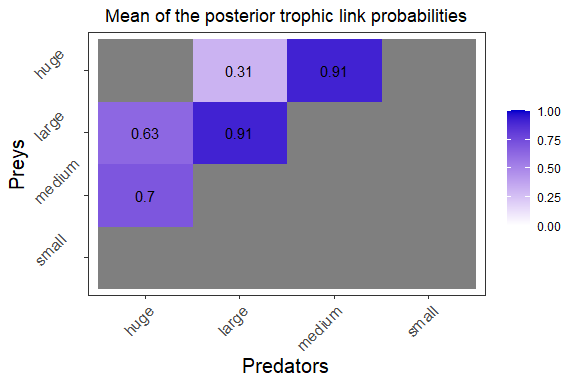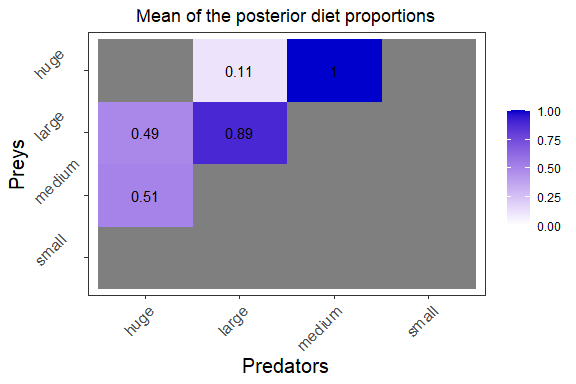You can access the main statistics by variable directly from the jagsUI object returned by the rrun_model function, including the mean value of the variables: print(mcmc_output_example$summary[,"mean"])
#>   eta[2,1]   eta[3,1]   eta[3,2]   eta[4,2]   eta[4,3]    PI[2,1]    PI[3,1]
#>  0.6989887  0.6298710  0.9065303  0.3095975  0.9057456  0.5103627  0.4896373
#>    PI[3,2]    PI[4,2]    PI[4,3]   deviance
#>  0.8851660  0.1148340  1.0000000 75.8304573

### The probability distributions

The probability distributions can be plotted for one predator:

plot_results(mcmc_output_example, data, pred = "huge")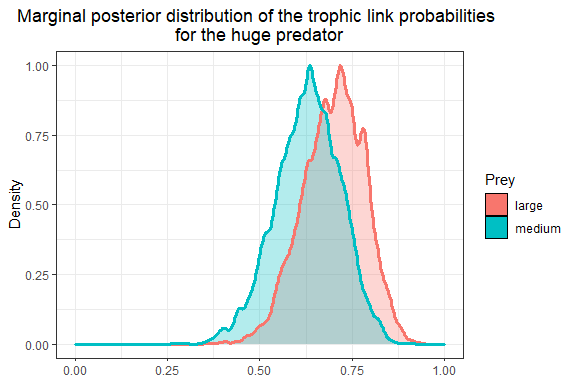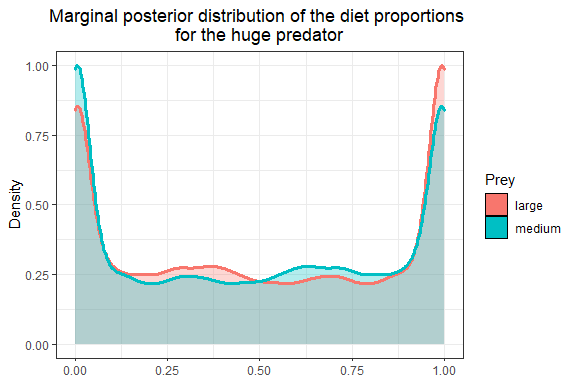plot_results(mcmc_output_example, data, pred = "large")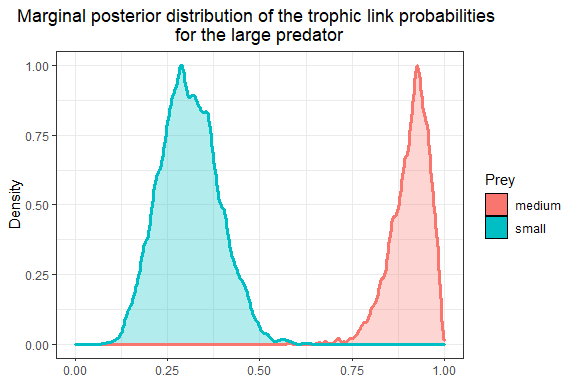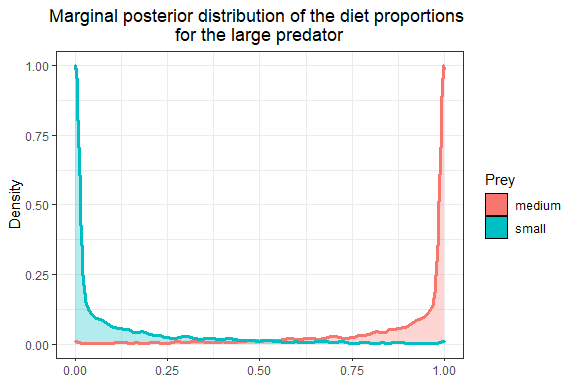## 7. Save another variable than $$\Pi$$ and $$\eta$$

You actually have the possibility to monitor and output the statistics of all the model parameters. For example you may be interested by the variable $$\delta$$ that represents the trophic discrimination factor. In the EcoDiet model, a different trophic discrimination factor is used for each trophic group and for each element, allowing some differences between species. We can chose to monitor these parameters when running the model by using the variables_to_save argument:

mcmc_output_delta <- run_model(filename, data,
variables_to_save = c("delta"),
run_param = "test")

And now you can access the mean value using:

print(mcmc_output_delta\$summary[,"mean"])